# Federgraph Formula

## The formula of Sample 1

The Federgraph application does not only plot Betrag, Richtung, and Energie, but also something like abs(u), even if this is just for fun. Sample 1 in the Federgraph application uses the formula shown in the following image.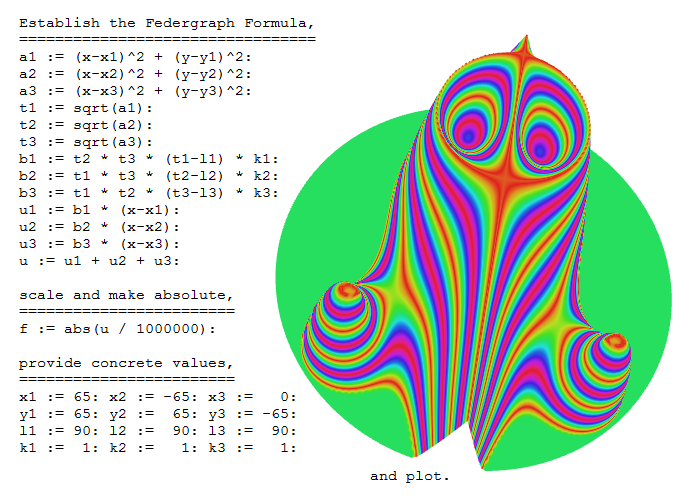The formula above is given in Maple syntax.

## Using an old version of Maple

The monument of Federgraph ancestors: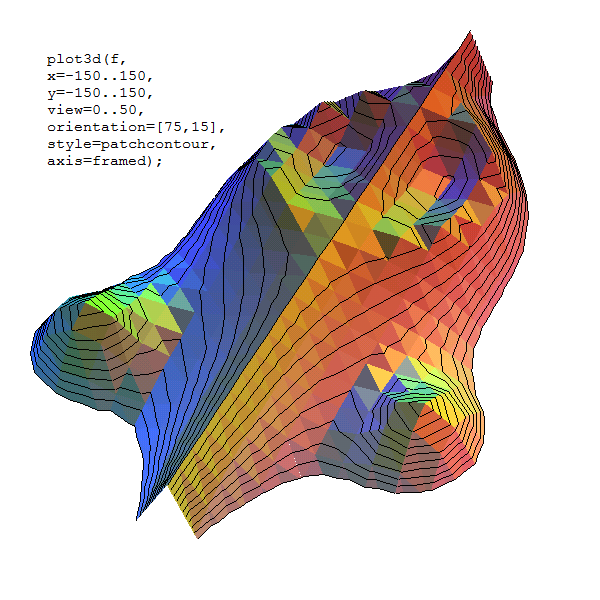The image shows that the sample can be recreated in the old 16 bit student version of Maple, which I used back in 1994.

## Using a current version of Maxima

Maxima is a free program you can use to prove it; just copy and paste the text out of the following preformatted paragraph into Maxima:

``````/* Establish the Federgraph formula */

a1: (x-x1)^2 + (y-y1)^2\$
a2: (x-x2)^2 + (y-y2)^2\$
a3: (x-x3)^2 + (y-y3)^2\$

t1: sqrt(a1)\$
t2: sqrt(a2)\$
t3: sqrt(a3)\$

b1: t2 * t3 * (t1-l1) * k1\$
b2: t1 * t3 * (t2-l2) * k2\$
b3: t1 * t2 * (t3-l3) * k3\$

u1: b1 * (x-x1)\$
u2: b2 * (x-x2)\$
u3: b3 * (x-x3)\$

u: u1 + u2 + u3\$

/* scale and make absolute */

f: abs(u / 1000000)\$

/* provide concrete values */

x1: 65\$ x2:-65\$ x3:  0\$
y1: 65\$ y2: 65\$ y3:-65\$
l1: 90\$ l2: 90\$ l3: 90\$
k1:  1\$ k2:  1\$ k3:  1\$

/* and plot */
plot3d(f, [x,-150,150], [y,-150,150],  [z,0,50],  [plot_format,gnuplot]);
``````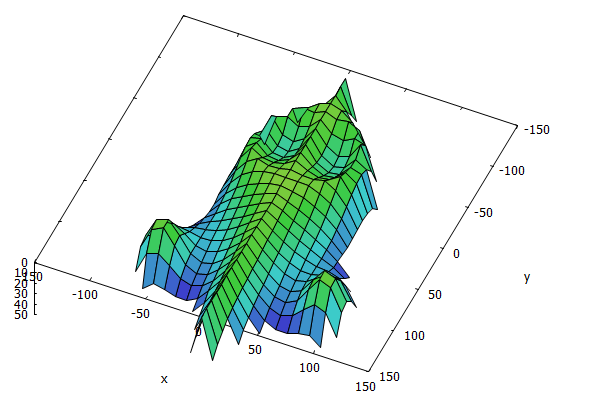The image above is a screenshot of the gnuplot output.

## Using Mathematica

This will work on the Raspberry Pi where Mathematica is a free download.

``````(* Clear *)
ClearAll[x1, x2, x3]
ClearAll[y1, y2, y3]
ClearAll[l1, l2, l3]
ClearAll[k1, k2, k3]

(* Establish the Federgraph formula *)
a1=(x-x1)^2+(y-y1)^2;
a2=(x-x2)^2+(y-y2)^2;
a3=(x-x3)^2+(y-y3)^2;

t1=Sqrt[a1];
t2=Sqrt[a2];
t3=Sqrt[a3];

b1=t2*t3*(t1-l1)*k1;
b2=t1*t3*(t2-l2)*k2;
b3=t1*t2*(t3-l3)*k3;

u1=b1*(x-x1);
u2=b2*(x-x2);
u3=b3*(x-x3);

u=u1+u2+u3;

(*scale and make absolute*)
f= Abs[u/1000000]

(*provide concrete values*)
x1=65;
x2=-65;
x3=0;
y1=65;
y2=65;
y3=-65;
l1=90;
l2=90;
l3=90;
k1=1;
k2=1;
k3=1;

(*and plot*)
Plot3D[f,{x,-150,150},{y,-150,150}, PlotRange -> {0, 50}]
``````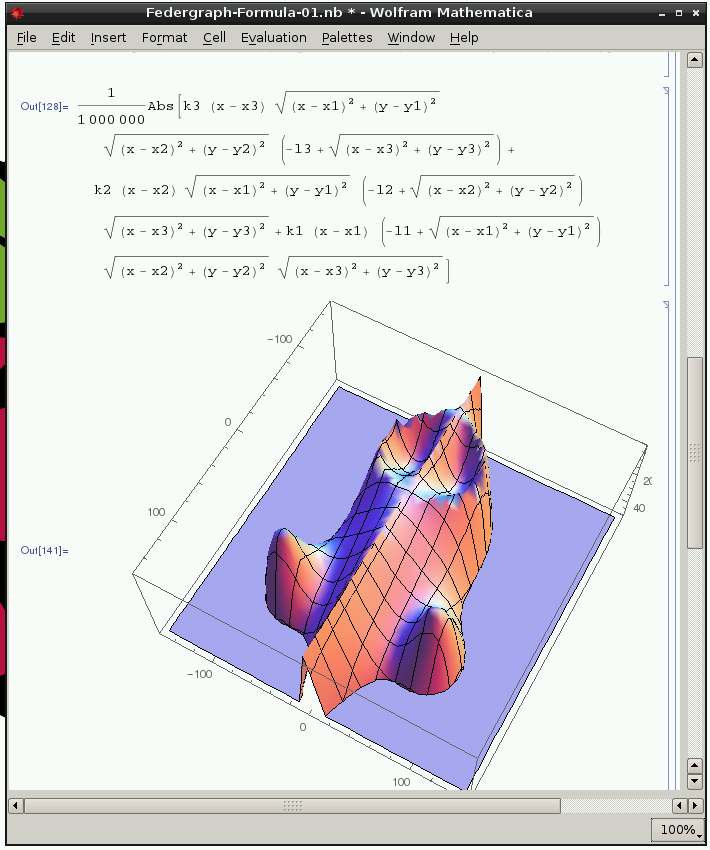## Using Sage

``````# provide concrete values
x1=65; x2=-65; x3=0
y1=65; y2= 65; y3=-65;
l1=90; l2= 90; l3= 90;
k1= 1; k2=  1; k3=  1;

# establish the Federgraph formula
x, y = var('x,y')

a1=(x-x1)^2+(y-y1)^2;
a2=(x-x2)^2+(y-y2)^2;
a3=(x-x3)^2+(y-y3)^2;

t1=sqrt(a1);
t2=sqrt(a2);
t3=sqrt(a3);

b1=t2*t3*(t1-l1)*k1;
b2=t1*t3*(t2-l2)*k2;
b3=t1*t2*(t3-l3)*k3;
u1=b1*(x-x1);
u2=b2*(x-x2);
u3=b3*(x-x3);

u=u1+u2+u3;

# scale and make absolute
f= abs(u/1000000)

def g(x, y):
temp = f(x, y)
if temp > 50:
temp = 50
if temp > 0:
temp = 0
return temp

# and plot
plot3d(g, (x,-150,150), (y,-150,150) )
``````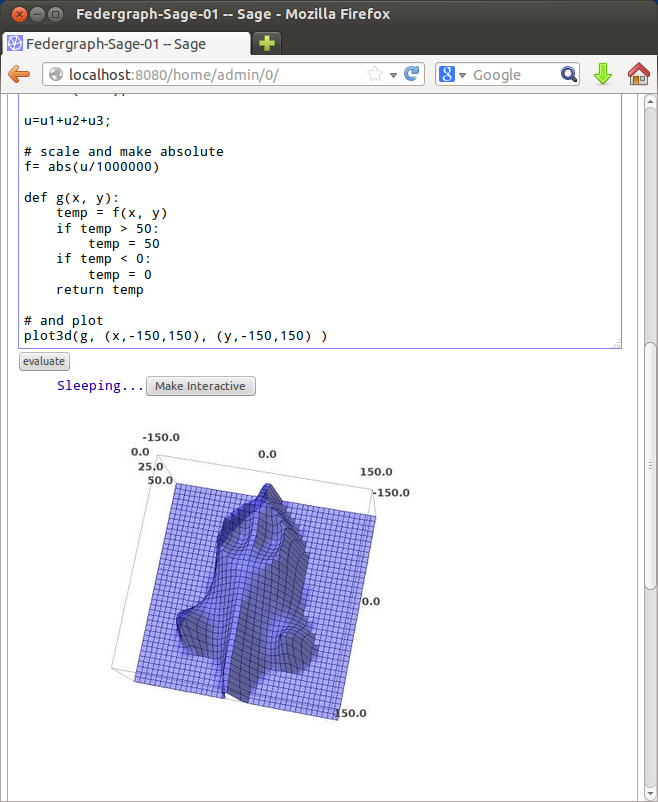## 21st century X-Eyes

If you change the texture parameters, Federgraph rolls with its eyes. It may be seen as a modern age (GPU intensive) alternative to X-Eyes, which you must see live!